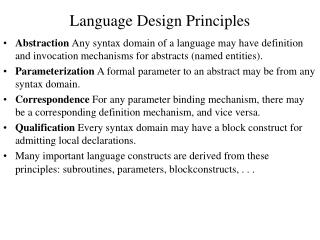DownloadDownload PresentationLanguage Design Principles

Language Design Principles

Télécharger la présentationLanguage Design Principles

- - - - - - - - - - - - - - - - - - - - - - - - - - - E N D - - - - - - - - - - - - - - - - - - - - - - - - - - -
Presentation Transcript

1. Language Design Principles • Abstraction Any syntax domain of a language may have definition and invocation mechanisms for abstracts (named entities). • Parameterization A formal parameter to an abstract may be from any syntax domain. • Correspondence For any parameter binding mechanism, there may be a corresponding definition mechanism, and vice versa. • Qualification Every syntax domain may have a block construct for admitting local declarations. • Many important language constructs are derived from these principles: subroutines, parameters, block­constructs, . . .

2. Abstraction Examples: • Specification that hides computational details - Abstract datatypes. • Function expressions (x:M ) • Named abstractions • Definition: square = (–n: n times n), • Invocation: square(two). • Procedures • Abstraction of a command. • Invoked by mentioning its name.

3. Abstractions • Definition mechanism. • Invocation mechanism. • Abstraction = name plus body • Body expression from syntax domain B. • Abstract can be invoked by using its name any place where a B expression is (syntactically) legal. • EXAMPLES: Command abstracts (procedures), Expression abstracts (functions, constants), Declaration abstracts (classes, modules), Type abstracts (named types). • Principle of Abstraction: Any syntax domain of a language may have definition and invocation mechanisms for abstracts.

4. Abstractions Abstract [[define I=V]]. • Name [[I]]. • Body [[V]]. • Denotable value V[[V]]. • Question: when is body evaluated? • At point of definition? • At point of invocation? • Fig 8.1

5. Command Abstracts Command abstracts = procedures. D ::= D1;D2 | var I:T | proc I=C C ::= C1;C2|L:=E | begin D;C end|I|… Denotation of abstract's body: • C[[C]]:Environment Store  Poststore • Environment and store are provided at the point of invocation (dynamic scoping). • (C[[C]]e): Store  Poststore  • Environment provided at the point of definition, store provided at point of invocation (static scoping). • (C[[C]]e s)  Poststore  • Procedure completely evaluated at point of definition and [[I ]] is bound to result store.

6. Command Abstracts: Static scoping. • Proc = Store  Poststore  • Denotable­value = Natlocn + Array + Record + Proc • D[[proc I=C]] = e. s.((updateenv [[I]] inProc(C[[C]]e) e), (return s)) • C[[I]] = e. s.cases (accessenv [[I]] e) of isNatlocn(l)  (signalerr s) . . . [] isProc(q)  (q s) end • C[[C]]e is bound to [[I]] in environment, store is supplied at invocation­time.

7. Expression Abstracts • Expression abstracts = functions. • D ::= ..|fcn I =E • E ::= E 1 +E 2|…|I • Constant definition. • Environment and store are bound at point of definition. • Function definition. • Only environment is bound at point of definition, store is supplied at invocation­time. • Macro definition. • Both environment and store are supplied at invocation­time. • Constants, functions, macros are variations of the same mechanism!

8. Function Procedures Hybrid of procedure and function. D ::= …| fcnproc I=C resultis E • Command abstract. • Invoked by an Expression­identifier. • Returns an expressible value. • Expression can alter the value of the store! • Function procedure may not terminate!

9. Function Procedures • E: Expression  Environment  Store  (Expressible­value x Poststore)  • D[[fcnproc I=C resultis E]] = e. s. ((updateenv [[I]] inFcn­proc((check (E[[E]]e)) o (C[[C]]e)) e), (return s)) • E[[I]] = e. s. cases (accessenv [[I]] e) of isNatlocn(l) ((access l s), (return s)) . . . [] isFcn­proc(f) ! (f s) end All semantic equations for E must be revised to cope with side­effects and non- termination!

10. Declaration Abstracts • D ::= D1;D2|var I:T|...|class I=D| I • Invocation of a class activates declarations in its body. • Multiple invocations of same class in a block lead to a redefinition error. • Renaming of declarations required. • Modules are similar to classes

11. Type Abstracts • D ::= . . . | type I=T • T ::= nat | . . . | I • D[[type I=T]] = e. s.((updateenv [[I]] inType(T[[T]]e) e), (return s)) • T: Type­structure  Environment  Store  (Denotable­value x Poststore) • T[[I]] = e. s. cases (accessenv [[I]] e) of isNatlocn(l) (inErrvalue(), (signalerr s)) ...[] isType(v)  (v s) end • When are two variables equivalent in type?

12. Type Equivalence • Structure equivalence. • Two variables are type­equivalent if they have identical storage structures. • Occurence equivalence (name equivalence). • Two variables are type­equivalent if they are defined with the same occurence of a type expression. • Structure equivalence more natural in denotational semantics.

13. Example type M = nat; type N = array [1..3] of M; var A: nat; var B: M; var C: M; var D: N; var E: array [2..4] of nat • A and B are structure­equivalent but not occurrence equiv. • B and C are both structure­ and occurence­equivalent. • C and D are neither structure­nor occurence­equivalent. • Are D and E structure­equivalent? • Different range bounds in environment. • Similar problems for record types.

14. Recursive Bindings D ::= …|rec abs I=M |... abs {proc,fun,class, …} D[[rec abs I=M]] = e. s(e’,(return s)) where e’ = (updateenv [[I]]inM(M[[M]]e’) e) Means for terminating recursive invocations. E ::= E 1 +E 2 |…| if E1 then E2 else E3 T ::= nat|…|if E then T1 else T2 Which constructions are chosen, depends on language pragmatics.